Next: Scattering Theory Up: Variational Methods Previous: Helium Atom

# Hydrogen Molecule Ion

The hydrogen molecule ion consists of an electron orbiting about two protons, and is the simplest imaginable molecule. Let us investigate whether or not this molecule possesses a bound state: i.e., whether or not it possesses a ground-state whose energy is less than that of a hydrogen atom and a free proton. According to the variation principle, we can deduce that theion has a bound state if we can find any trial wavefunction for which the total Hamiltonian of the system has an expectation value less than that of a hydrogen atom and a free proton.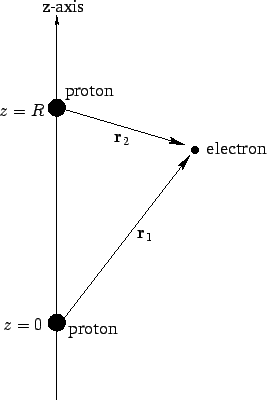Suppose that the two protons are separated by a distance. In fact, let them lie on the-axis, with the first at the origin, and the second at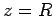(see Fig. 26). In the following, we shall treat the protons as essentially stationary. This is reasonable, since the electron moves far more rapidly than the protons.

Let us try(1223)

as our trial wavefunction, where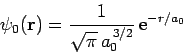(1224)

is a normalized hydrogen ground-state wavefunction centered on the origin, and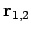are the position vectors of the electron with respect to each of the protons (see Fig. 26). Obviously, this is a very simplistic wavefunction, since it is just a linear combination of hydrogen ground-state wavefunctions centered on each proton. Note, however, that the wavefunction respects the obvious symmetries in the problem.

Our first task is to normalize our trial wavefunction. We require that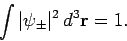(1225)

Hence, from (1223),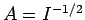, where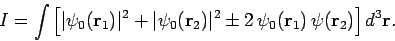(1226)

It follows that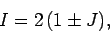(1227)

with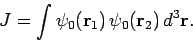(1228)

Let us employ the standard spherical polar coordinates (,,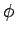). Now, it is easily seen thatand. Hence,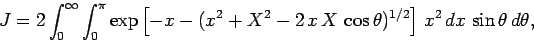(1229)

where. Here, we have already performed the trivialintegral. Let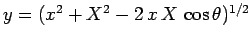. It follows that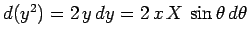, giving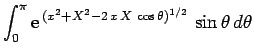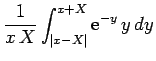(1230)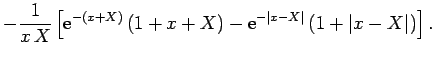Thus,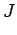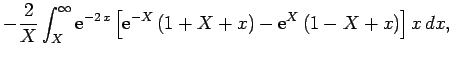(1231)

which evaluates to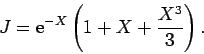(1232)

Now, the Hamiltonian of the electron is written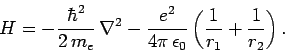(1233)

Note, however, that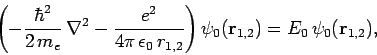(1234)

since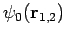are hydrogen ground-state wavefunctions. It follows that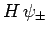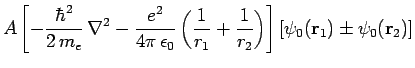(1235)

Hence,(1236)

where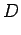(1237)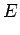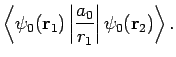(1238)

Now,(1239)

which reduces to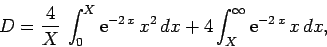(1240)

giving(1241)

Furthermore,(1242)

which reduces to(1243)

yielding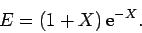(1244)

Our expression for the expectation value of the electron Hamiltonian is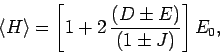(1245)

where,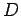, andare specified as functions ofin Eqs. (1232), (1241), and (1244), respectively. In order to obtain the total energy of the molecule, we must add to this the potential energy of the two protons. Thus,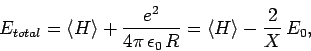(1246)

since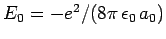. Hence, we can write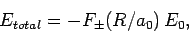(1247)

whereis the hydrogen ground-state energy, and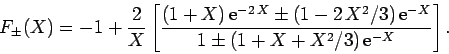(1248)

The functions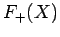and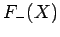are both plotted in Fig. 27. Recall that in order for theion to be in a bound state it must have a lower energy than a hydrogen atom and a free proton: i.e.,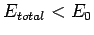. It follows from Eq. (1247) that a bound state corresponds to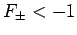. Clearly, the even trial wavefunctionpossesses a bound state, whereas the odd trial wavefunction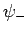does not [see Eq. (1223)]. This is hardly surprising, since the even wavefunction maximizes the electron probability density between the two protons, thereby reducing their mutual electrostatic repulsion. On the other hand, the odd wavefunction does exactly the opposite. The binding energy of theion is defined as the difference between its energy and that of a hydrogen atom and a free proton: i.e.,(1249)

According to the variational principle, the binding energy is less than or equal to the minimum binding energy which can be inferred from Fig. 27. This minimum occurs when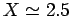and. Thus, our estimates for the separation between the two protons, and the binding energy, for theion areandeV, respectively. The experimentally determined values are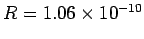m, and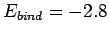eV, respectively. Clearly, our estimates are not particularly accurate. However, our calculation does establish, beyond any doubt, the existence of a bound state of theion, which is all that we set out to achieve.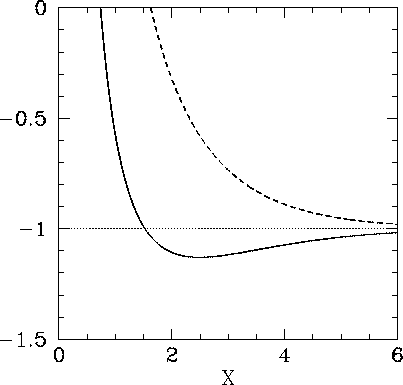Next: Scattering Theory Up: Variational Methods Previous: Helium Atom
Richard Fitzpatrick 2010-07-20